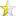• Articles
• The Conditional (or Ternary) Operator (?
Sep 23, 2009 (last update: Apr 16, 2013)

# The Conditional (or Ternary) Operator (?:)Introduction

The conditional operator is an operator used in C and C++ (as well as other languages, such as C#). The ?: operator returns one of two values depending on the result of an expression.

Syntax

`(expression 1) ? expression 2 : expression 3`
If expression 1 evaluates to true, then expression 2 is evaluated.

If expression 1 evaluates to false, then expression 3 is evaluated instead.

Examples

`#define MAX(a, b) (((a) > (b)) ? (a) : (b)) `
In this example, the expression `a > b` is evaluated. If it evaluates to true then a is returned. If it evaluates to false, b is returned. Therefore, the line `MAX(4, 12);` evaluates to 12.

You can use this to pick which value to assign to a variable:
`int foo = (bar > bash) ? bar : bash;`
In this example, either 'bar' or 'bash' is assigned to 'foo', depending on which is bigger.

Or even which variable to assign a value to:
`((bar > bash) ? bar : bash) = foo;`
Here, 'foo' is assigned to 'bar' or 'bash', again depending on which is bigger.

Caveats
The most likely mistake you may make while using the conditional operator is to forget operator precedence. The ?: operator has a fairly low precedence and as such it is easy to make various mistakes.

See http://www.difranco.net/cop2220/op-prec.htm for a table of C++ operators and their precedence (it is in descending order,).